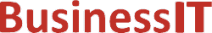O nás     Inzerce     KontaktSpolehlivé informace o IT již od roku 2011[Linux manuál]

# fpclassify, isfinite, isnormal, isnan: Makra s pohyblivou řádovou čárkou

Originální popis anglicky: fpclassify, isfinite, isnormal, isnan - floating-point classification macros

Návod, kniha: Linux Programmer's Manual

## STRUČNĚ

```#include <math.h>

int fpclassify(x);

int isfinite(x);

int isnormal(x);

int isnan(x);

int isinf(x);
```

## POPIS / INSTRUKCE

Floating point numbers can have special values, such as infinite or NaN. With the macro fpclassify(x) you can find out what type x is. The macro takes any floating-point expression as argument. The result takes one of the following values:
FP_NAN
x is "Not a Number".
FP_INFINITE
x is either plus or minus infinity.
FP_ZERO
x is zero.
FP_SUBNORMAL
x is too small to be represented in normalized format.
FP_NORMAL
if nothing of the above is correct that it must be a normal floating-point number.
The other macros provide a short answer to some standard questions.
isfinite(x)
returns a nonzero value if

(fpclassify(x) != FP_NAN && fpclassify(x) != FP_INFINITE)
isnormal(x)
returns a nonzero value if (fpclassify(x) == FP_NORMAL)
isnan(x)
returns a nonzero value if (fpclassify(x) == FP_NAN)
isinf(x)
returns a nonzero value if (fpclassify(x) == FP_INFINITE)

## NOTE

On systems conforming to BSD 4.3, isinf() will return 1 for positive, and -1 for negative infinity.

C99

## SOUVISEJÍCÍ

finite(3), INFINITY(3), isgreater(3)

 2004-10-31
©2011-2020 BusinessIT.cz, ISSN 1805-0522 | Názvy použité v textech mohou být ochrannými známkami příslušných vlastníků.
Provozovatel: Bispiral, s.r.o., kontakt: BusinessIT(at)Bispiral.com | Inzerce: Best Online Media, s.r.o., zuzana@online-media.cz
O vydavateli | Pravidla webu BusinessIT.cz a ochrana soukromí | pg(8925)